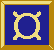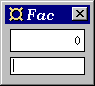## A program to test the factorial function

### Version 1

This program illustrates how data is communicated between different parts of a Fudget program. It illustrates a simple way to combine application specific code (in this case the factorial function) with GUI elements from the Fudget library.The program shows a numeric entry field at the bottom and a number display at the top. Whenever the user enters a number in the entry field and presses Return the factorial of that number is computed and displayed in the number display.

Here is the source code:

```import Fudgets

main = fudlogue (shellF "Fac" facF)

facF = intDispF >==< mapF fac >==< intInputF

fac 0 = 1
fac n = n * fac (n-1)
```

### Things to note

• The program (`facF`) is structured as a serial composition of three parts, using the operator `>==<`, Notice that data flow from right to left. The parts are:
• the numeric entry field `intInputF`,
• `mapF fac`, an abstract fudget that applies `fac`, the factorial function, to integers received from the entry field, and
• the number display `intDispF` which displays the factorials.

• We have used `fudlogue` and `shellF` on the top level as in the previous examples.

• Next Example
• Previous Example
• Back to the Example list